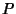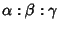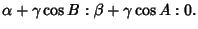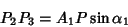## Pedal TriangleGiven a point, the pedal triangle ofis the Triangle whose Vertices are the feet of the perpendiculars fromto the side lines. The pedal triangle of a Triangle with Trilinear Coordinatesand angles,, andhas Vertices with Trilinear Coordinates(1)(2)(3)

The third pedal triangle is similar to the original one. This theorem can be generalized to: theth pedal-gon of any-gon is similar to the original one. It is also true that(4)

(Johnson 1929, pp. 135-136). The Areaof the pedal triangle of a pointis proportional to the Power ofwith respect to the Circumcircle,(5)

(Johnson 1929, pp. 139-141).

See also Antipedal Triangle, Fagnano's Problem, Pedal Circle, Pedal Line, Schwarz's Triangle Problem

References

Coxeter, H. S. M. and Greitzer, S. L. Geometry Revisited. Washington, DC: Math. Assoc. Amer., pp. 22-26, 1967.

Johnson, R. A. Modern Geometry: An Elementary Treatise on the Geometry of the Triangle and the Circle. Boston, MA: Houghton Mifflin, 1929.